Previous: Shape interface, Up: Interface reference

#### 8.2.11 Transformation interface

The transformation interface is an interface for container nodes which change the coordinate system of their children. It has several public methods.

Every node in the VCL node tree has its own coordinate space. A node implementing the transformation interface has two different spaces – the standard obj's coordinate space and the children coordinate space (which is equivalent to the coordinate space of its children). Between these two spaces there are transformations given by transformation matrices. The primary matrix is from children space to obj's space and the inverted matrix is from obj's space to children space.

Every object implementing the placement interface must also implement the object interface, the node interface and the container interface.

Transformation methods

The `_apply_point` methods just convert `struct geom_point` from one space to another. The `_matrix` methods are more complicated. They take a transformation as an argument and merge it with the primary or inverted transformation. Suppose that O represents the obj's space, C represents the children's space, X represents another space and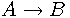represents the transformation matrix from A to B. So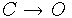is the primary matrix and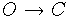is the inverted matrix. The appropriate `_matrix` methods can be described in the following way:

`_primary_apply_matrix`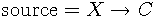,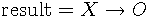`_inverted_apply_matrix`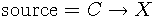,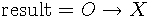`_primary_preply_matrix`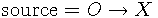,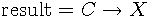`_inverted_preply_matrix`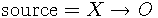,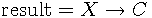The return values of these functions are error values (0 = OK) with the same meaning as the standard error values of GEOMLIB.

```     int vcl_transformation_primary_apply_matrix (void * obj,
const struct geom_transform *src, struct geom_transform *dst)

int vcl_transformation_inverted_apply_matrix (void * obj,
const struct geom_transform *src, struct geom_transform *dst)